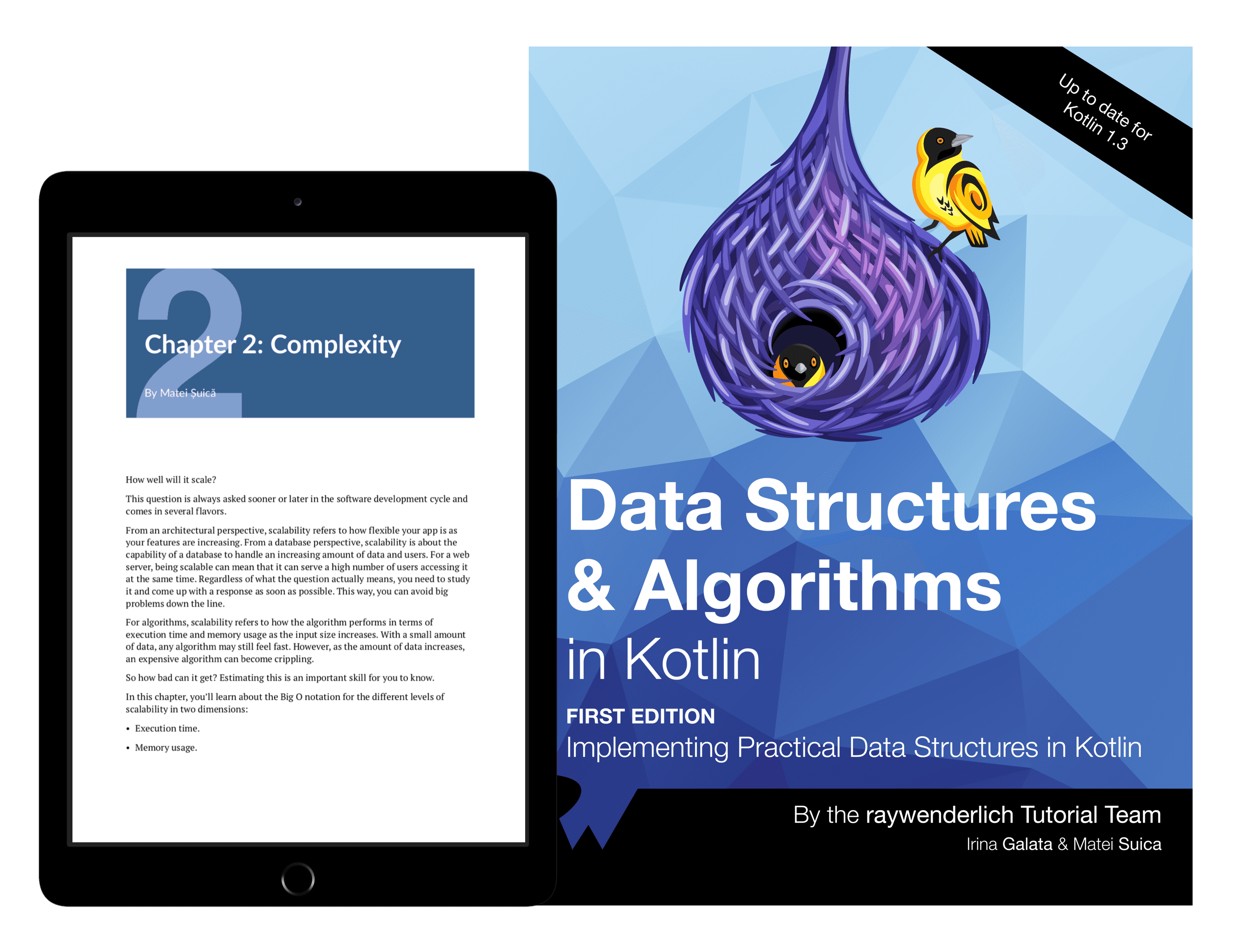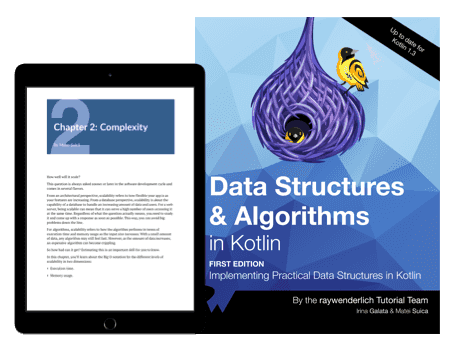# Data Structures & Algorithms in Kotlin

\$59.99 4.2/5 5 reviews · Write a Review
• Platform Android 10
• Language Kotlin 1.3
• Editor IDEA

A book that teaches you the fundamental tools of implementing key data structures in Kotlin, and how to use them to solve algorithms.

Developer Guide

### Intermediate## Learn Data Structures & Algorithms in Kotlin!

Data structures and algorithms are fundamental tools every developer should have. In this book, you'll learn how to implement key data structures in Kotlin, and how to use them to solve a robust set of algorithms.

This book is for intermediate Kotlin or Android developers who already know the basics of the language and want to improve their knowledge.

### Topics Covered in This Book

• Introduction to Kotlin: If you're new to Kotlin, you can learn the main constructs and begin writing code.
• Complexity: When you study algorithms, you need a way to compare their performance in time and space. Learn about the Big-O notation to help you do this.
• Elementary Data Structures: Learn how to implement Linked List, Stacks, and Queues in Kotlin.
• Trees: Learn everything you need about Trees — in particular, Binary Trees, AVL Trees, as well as Binary Search and much more.
• Sorting Algorithms: Sorting algorithms are critical for any developer. Learn to implement the main sorting algorithms, using the tools provided by Kotlin.
• Graphs: Have you ever heard of Dijkstra and the calculation of the shortest path between two different points? Learn about Graphs and how to use them to solve the most useful and important algorithms.

### Section I: Introduction to Data Structures & Algorithms

1.

#### Kotlin & Kotlin Standard Library

The Kotlin Standard Library refers to the framework that defines the core elements of the Kotlin language. Inside the Kotlin Standard Library, you’ll find a variety of tools and data types to help build your Kotlin apps, including data structures.

2.

#### Complexity

Answering the question, “Does it scale?” is all about understanding the complexity of an algorithm. The Big-O notation is the primary tool that you’ll use to think about algorithmic performance in the abstract and independent hardware or language. This chapter will prepare you to think in these terms.

### Section II: Elementary Data Structures

3.

A linked list is a collection of values arranged in a linear, unidirectional sequence. A linked list has several theoretical advantages over contiguous storage options such as the array, including constant time insertion and removal from the front of the list, and other reliable performance characteristics.

4.

#### Stack Data Structures

The stack data structure is identical in concept to a physical stack of objects. When you add an item to a stack, you place it on top of the stack. When you remove an item from a stack, you always remove the topmost item. Stacks are useful, and also exceedingly simple. The main goal of building a stack is to enforce how you access your data.

5.

#### Queues

Lines are everywhere, whether you’re lining up to buy tickets to your favorite movie or waiting for a printer machine to print out your documents. These real-life scenarios mimic the queue data structure. Queues use first in, first out ordering. In other words, the first element that was enqueued will be the first to get dequeued. Queues are handy when you need to maintain the order of your elements to process later.

### Section III: Trees

6.

#### Trees

The tree is a data structure of profound importance. It’s used to tackle many recurring challenges in software development such as representing hierarchical relationships, managing sorted data and facilitating fast lookup operations. There are many types of trees, and they come in various shapes and sizes.

7.

#### Binary Trees

In the previous chapter, you looked at a basic tree where each node can have many children. A binary tree is a tree where each node has at most two children, often referred to as the left and right children. Binary trees serve as the basis for many tree structures and algorithms. In this chapter, you’ll build a binary tree and learn about the three most important tree traversal algorithms.

8.

#### Binary Search Trees

A binary search tree facilitates fast lookup, addition and removal operations. Each operation has an average time complexity of O(log n), which is considerably faster than linear data structures such as arrays and linked lists.

9.

#### AVL Trees

In the previous chapter, you learned about the O(log n) performance characteristics of the binary search tree. However, you also learned that unbalanced trees can deteriorate the performance of the tree, all the way down to O(n). In 1962, Georgy Adelson-Velsky and Evgenii Landis came up with the first self-balancing binary search tree: the AVL Tree.

10.

#### Tries

The trie (pronounced as “try”) is a tree that specializes in storing data that can be represented as a collection, such as English words. The benefits of a trie are best illustrated by looking at it in the context of prefix matching, which is what you’ll do in this chapter.

11.

#### Binary Search

Binary search is one of the most efficient searching algorithms with a time complexity of O(log n). This is comparable with searching for an element inside a balanced binary search tree. To perform a binary search, the collection must be able to perform index manipulation in constant time and must be sorted.

12.

#### The Heap Data Structure

A heap is a complete binary tree, also known as a binary heap, that can be constructed using an array. Heaps come in two flavors: Max heaps and Min heaps. Have you seen the movie Toy Story with the claw machine and the squeaky little green aliens? Imagine that the claw machine is operating on your heap structure and will always pick the minimum or maximum value depending on the flavor of heap.

13.

#### Priority Queues

Queues are lists that maintain the order of elements using first in, first out (FIFO) ordering. A priority queue is another version of a queue that, instead of using FIFO ordering, dequeues elements in priority order. A priority queue is especially useful when you need to identify the maximum or minimum value given a list of elements.

### Section IV: Sorting Algorithms

14.

#### O(n²) Sorting Algorithms

O(n²) time complexity doesn’t have great performance, but the sorting algorithms in this category are easy to understand and useful in some scenarios. These algorithms are space-efficient and only require constant O(1) additional memory space. In this chapter, you’ll look at the bubble sort, selection sort and insertion sort algorithms.

15.

#### Merge Sort

In this chapter, you’ll study one of the most important sorting algorithm based on the divide and conquer principle. You’ll learn how to split an array, sort it recursively and then merge the two parts together.

16.

So far, you’ve been relying on comparisons to determine the sorting order. In this chapter, you’ll look at a completely different model of sorting. Radix sort is a non-comparative algorithm for sorting integers in linear time. There are multiple implementations of radix sort that focus on different problems. To keep things simple, you’ll focus on sorting base 10 integers while investigating the least significant digit (LSD) variant of radix sort.

17.

#### Heapsort

Heapsort is another comparison-based algorithm that sorts an array in ascending order using a heap. This chapter builds on the heap concepts presented in Chapter 12, “The Heap Data Structure.” Heap sort takes advantage of a heap being, by definition, a partially sorted binary tree.

18.

#### Quicksort

Quicksort is another divide-and-conquer technique that introduces the concept of partitions and a pivot to implement high-performance sorting. You‘ll see that while it’s extremely fast for some datasets, for others, it can be a bit slow.

### Section V: Graphs

19.

#### Graphs

What do social networks have in common with booking cheap flights around the world? You can represent both of these real-world models as graphs. A graph is a data structure that captures relationships between objects. It’s made up of vertices connected by edges. In a weighted graph, every edge has a weight associated with it that represents the cost of using this edge. This lets you choose the cheapest or shortest path between two vertices.

20.

In the previous chapter, you explored how graphs can be used to capture relationships between objects. Several algorithms exist to traverse or search through a graph’s vertices. One such algorithm is the breadth-first-search algorithm, which you can use to solve a wide variety of problems, including generating a minimum spanning tree, finding potential paths between vertices and finding the shortest path between two vertices.

21.

#### Depth-First Search

In the previous chapter, you looked at breadth-first-search, where you had to explore every neighbor of a vertex before going to the next level. In this chapter, you’ll look at depth-first search, which has applications for topological sorting, detecting cycles, pathfinding in maze puzzles and finding connected components in a sparse graph.

22.

#### Dijkstra’s Algorithm

Have you ever used the Google or Apple Maps app to find the shortest or fastest from one place to another? Dijkstra’s algorithm is particularly useful in GPS networks to help find the shortest path between two places. Dijkstra’s algorithm is a greedy algorithm that constructs a solution step-by-step and picks the most optimal path at every step.

23.

#### Prim's Algorithm

In previous chapters, you looked at depth-first and breadth-first search algorithms. These algorithms form spanning trees. In this chapter, you’ll look at Prim’s algorithm, a greedy algorithm used to construct a minimum spanning tree. A minimum spanning tree is a spanning tree with weighted edges where the total weight of all edges is minimized. You’ll learn how to implement a greedy algorithm to construct a solution step-by-step and pick the most optimal path at every step.

4.2 /5

### Leave a review for this product

5 reviews## Data Structures & Algorithms in Kotlin

A book that teaches you the fundamental tools of implementing key data structures in Kotlin, and how to use them to solve algorithms.

Top
&nbsp;